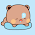Saturday, May 15, 2010

Infix to Postfix transformation and evaluation

Here, I would like to share a java source for converting an Infix expression to a Postfix equivalent and evaluate the Postfix expression. Postfix is also known as "Reverse Polish Notation". If you want to know more about this algorithm, this will be helpful.

Here is a simple java implementation. (Oh, we could do it a lot easily in C++, but, actually it has a academic purpose as well). A few things to note:
• Fixed format of input, as this is just a demonstration. Do not use spaces.
• It doesn't check whether the given expression is consistent or not.
• No math error is checked here, you have to add it to your own.
• Check sample execution for more details.
• Only for binary operators +,-,*,/,%,^ and parenthesis ()

Java Source

`//// @author Zobayer// @date May 10, 2010//import java.io.BufferedReader;import java.io.InputStreamReader;import java.io.IOException;import java.util.List;import java.util.ArrayList;import java.util.Stack;//// Demonstrates Expression evaluation process.// Doesn't take care of wrong input,// You need to handle that on your own.//public class Expression {    // A sample main() method to demonstrate this process    public static void main(String[] args) throws IOException {        BufferedReader stdin = new BufferedReader(new InputStreamReader(System.in));        String expr;        List<String> inFix, postFix;        int result;        while((expr = stdin.readLine())!=null) {            expr = "(" + expr + ")";            inFix = getInFix(expr);            postFix = getPostFix(inFix);            result = evaluate(postFix);            System.out.println("Postfix form: " + postFix);            System.out.println("Result: " + result);        }    }    // Parse the input string and form an infix notation    static List<String> getInFix(String expr) {        List<String> list = new ArrayList<String>();        int n, i;        char ch;        boolean hasInt;        for(i = n = 0, hasInt = false; i < expr.length(); i++) {            ch = expr.charAt(i);            if(!isDigit(ch)) {                if(hasInt) {                    list.add("" + n);                    n = 0;                    hasInt = false;                }                list.add("" + ch);            }            else {                n = n * 10 + (ch - 48);                hasInt = true;            }        }                return list;    }    // Enlist the tokens in a postfix notation    static List<String> getPostFix(List<String> inFix) {        List<String> list = new ArrayList<String>();        Stack<String> oper = new Stack<String>();        int i;        char ch;        String token, peek;        for(i = 0; i < inFix.size(); i++) {            token = inFix.get(i);            ch = token.charAt(0);            if(isDigit(ch)) list.add(token);            else if(ch=='(') oper.push("" + ch);            else if(ch==')') {                while(!oper.empty()) {                    peek = oper.pop();                    if(peek.charAt(0)!='(') list.add(peek);                    else break;                }            }            else {                while(!oper.empty()) {                    peek = oper.peek();                    if(peek.charAt(0)!='(' && preced(ch) <= preced(peek.charAt(0))) {                        list.add(peek);                        oper.pop();                    }                    else {                        oper.push(token);                        break;                    }                }            }        }                return list;    }    // Evaluate the postfix notation passed as a list    static int evaluate(List<String> postFix) {        Stack<Integer> stack = new Stack<Integer>();        int i, a, b;        String token;        char ch;        for(i = 0; i < postFix.size(); i++) {            token = postFix.get(i);            ch = token.charAt(0);            if(isDigit(ch)) stack.push(Integer.parseInt(token));            else {                b = stack.pop();                a = stack.pop();                switch(ch) {                    case '+': a = a + b; break;                    case '-': a = a - b; break;                    case '*': a = a * b; break;                    case '/': a = a / b; break;                    case '%': a = a % b; break;                    case '^': a = (int)Math.pow(a, b); break;                }                stack.push(a);            }        }                return stack.pop();    }    // Provides operator precedence    static int preced(char op) {        if(op=='^') return 3;        if(op=='*' || op=='/' || op=='%') return 2;        if(op=='+' || op=='-') return 1;        return 0;    }    // Checks if ch is a digit or not    static boolean isDigit(char ch) {        return (ch >= '0' && ch <= '9');    }}`

Sample run

`(3+8-90*36)*((((89-5%6+2^3-10-10-10)))-8)+100Postfix form: [3, 8, +, 90, 36, *, -, 89, 5, 6, %, -, 2, 3, ^, +, 10, -, 10, -, 10, -, 8, -, *, 100, +]Result: -17426690+0Postfix form: [90, 0, +]Result: 906+763-67*2367-(54/234)Postfix form: [6, 763, +, 67, 2367, *, -, 54, 234, /, -]Result: -157820`

The algorithm implemented here is pretty simple, so I guess I haven't made a mistake yet, but who knows? So please check it...

1.and yes, java sux

1.I am rolling on the floor now seeing you are a professional on java platform for two years in a row now.

2.Yeah, true... ROFLMAO

2.would be better if you use BigInteger, StringTokenizer. also you can seprate the main function from the Expression class and use non static methods.
you can also use
"for (String token : inFix) {"
The code looks like a complete machine translation from c++ code to java.
And definitely java doesn't su*k if you know how to use it.

3.yeah, you are right, but actually it was a lab work, and I didn't use StringTokenizer class intentionally, as, most of the students still don't know how to read input in java
but what I think, java is good for huge projects, not for implementing data structures which are built ins in almost all high level languages, in these case, the coding sux as its worthless.

4.my name is Dan by the way..

5.@Dan, at the bottom of the page...

6.I got the working code from http://code.google.com/p/expressionoasis/

7.Hmm, hey people, don't try to compare this with any professional piece, cause, as he has already mentioned, it's a school homework... So I should say, nice work...
--Kelvin